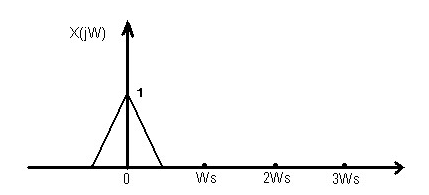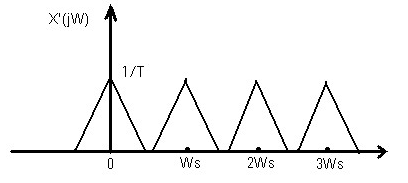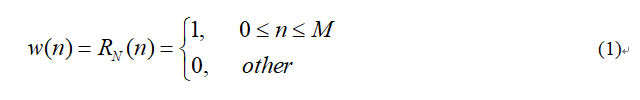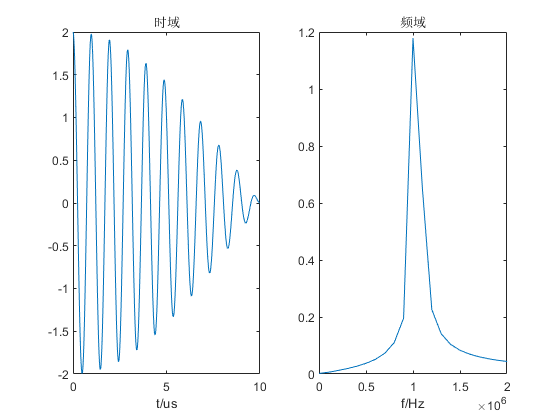• 信号x长度为Ts，通过傅氏变换后得到X，其频率分辨率为Δf=1/T（Hz），若经过采样后，假设采样频率为fs=1/Ts，而进行频谱分析时要将这个无穷长的序列使用窗函数截断处理，假设使用矩形窗，我们知道，矩形窗的频谱为...matlab
• ft_spect（2.0 版）计算具有所需频率分辨率的输入信号的幅度和相位谱，并过滤相位谱以抑制浮动舍入误差。 注意#1：ft_spect 不能消除频谱泄漏。 注意#2：离散傅立叶变换 (DFT) 将输入信号视为周期信号的一个周期，...matlab

简单来说，补零(Zero Padding)就是对变换前的时域或空域信号的尾部添加若干个0，以增加数据长度。如图1所示，为含有1.00 MHz 和1.05 MHz 两个频率成分合成的正弦波实信号。(a)(b)

图1 时域信号的补零示意图

图1(a)中信号长度为1000个样点，采样频率为fs=100 MHz时，信号的实际时长则为10 us。在其尾部添加1000个0，即数据增加到了2000个点（时长为20us），则变为图1(b)所示的波形。

三、关于FFT频率分辨率

这里涉及到两种意义下的分辨率问题，一种叫“波形频率分辨率（Waveform frequency resolution”）或叫视觉频率分辨率（Visual frequency resolution）；另一种则叫做“FFT分辨率”。虽然，这个分类和命名不一定是很专业的术语，但却有助于对“频率分辨率”概念的理解。在没有补零的情况下，这两个概念通常容易被混淆，因为它们是等价的。

波形频率分辨率是指可以被分辨的2个频率的最小间隔（Spacing）；而FFT 分辨率则是频谱中的数据点数（The number of points in the spectrum），它是与做FFT的点数直接相关的。

因此，波形频率分辨率可定义为：

ΔRw= 1/T 7

其中，T是实际信号的时间长度。

同样，FFT分辨率可以定义为：

ΔRf= fs/Nfft

其中，fs为采样频率（the sampling frequency），Nfft为FFT的点数。ΔRf代表了FFT频率轴上的频率取值的间隔（Spacing）。

值得注意的是，可能有很好的FFT分辨率，但却不一定能够很好的把2个频率成分简单的分开。同样，可能有很高的波形分辨率，但波形的能量峰值会通过整个频谱而分散开（这是因为FFT的频率泄漏现象）。

我们知道，信号的离散傅里叶变换（DFT）或快速傅里叶变换（FFT）是对波形的任何一边补零形成的无限序列进行计算的。这就是，为什么FFT的每个频率单元（bin）都具有明显的sinc 波的形状。

波形频率分辨率1/T与一个sinc函数空值间隔（the space between nulls）是一样的。

四、例析

x = sin(2*pi*1000000*t)+sin(2*pi*1050000*t)

其中，f1 = 1.00MHz，f2 = 1.05MHz，频率间隔为0.05MHz。也就是说，在我们的频谱分析曲线上能看到2个频率点的峰，若2个正弦波的幅度为1伏( V)，那么我们期望在1 MHz 和 1.05 MHz的频率点处的功率为10 dBm。

分以下几种情况进行分析：

1)时域信号1000个点采样，做相同样点数的FFT图2 原始信号的功率谱（1000点 FFT）

图2中，我们并没有看见期望的两个脉冲，因为图中仅出现一个脉冲点，其幅度约为11.4 dBm。显然，这个图并不是我们想要的正确的频谱图。原因很简单，没有足够的分辨率看见两个峰值（Peaks）。

2)时域信号1000个点采样，后端补6000个零，做7000点数的FFT

我们自然想到，采用补零方式增加FFT点数，以使频率轴上能增加更多点数。如采用7000个点做FFT，即需要在原1000点信号尾部增加6000个零值（即60us时长），则原始信号变为图3(a)所示，其FFT结果如图3(b)所示。(a)(b)

图3 原始信号补零及功率谱（7000点 FFT）

图3中，我们也并没有看见期望的结果。仔细观察一下，此图到底告诉了我们什么呢？即通过增加更多FFT点数的做法，使得波形频率分辨率公式中的sinc函数的定义更清晰。可以看出，sinc 空值（nulls）间隔大约是0.1 MHz。

再来看一下频率分辨率ΔRf告诉了我们什么。尽管，FFT分辨率大约为14kHz（足够的频率分辨率）， 而波形频率分辨率仅仅为100 kHz。两个信号的频率间隔是50kHz，所以我们受限于波形频率分辨率ΔRw。

3)时域信号7000个点采样，做7000点数的FFT

为了合理地解决这个频谱的问题，需要增加用于FFT的时域数据的长度（点数）。因此，我们直接采集波形的7000点作为输入信号，取代补零（Zero Padding）方式到 70us (7000 点) 。时间域信号及对应的功率谱分别如图4a-4b所示。(a)(b)

图4 按7000点采集的信号及其功率谱

通过时域数据的周期延拓，现在的波形频率分辨率ΔRw也近似为14KHz。但从频谱图中，我们还是看不见2个正弦波。1 MHz 信号已按正确的10 dBm功率值清晰地表征，而1.05 MHz 信号变宽，且未以期望的10 dBm 功率分布。这是为什么呢？

原因就是1.05 MHz处并没有FFT点的分布，原因是此处的能量被多个FFT点分散（泄露）了。

给出的例子中，采样频率是100 MHz，FFT点数为7000。频谱图中，点与点之间的间隔是14.28 kHz。1 MHz频率刚好为频率间隔的整数陪，而1.05 MHz 却不是。距1.05 MHz最近的整数倍频率为1.043 MHz 和1.057 MHz, 因此，能量被这2个FFT单元所分散。

4)时域信号7000个点采样，后端补1000个零，做8000点数的FFT。

为了解决这个问题，我们可以合理选择FFT的点数，以便这两个点能在频率轴上成为独立分开的点。由于，我们并不需要更好的波形频率分辨率，仅采用时域数据的零填充方式来调整FFT数据点的频率间隔。

给时域信号增加1000零值(10 us)，使得频率间隔为12.5 kHz，这样，满足了1 MHz and 1.05 MHz两个频率都是这个间隔的整数倍。此时，给出的功率谱如图5所示。可以看出，两个频率问题得到解决，而且功率均在期望的10 dBm。图5 补零至8000点信号的功率谱

为了进一步观察过度补零的现象，通过时域补更多的零值（10000点）来完成更多点数的FFT（确保具有正确的波形频率分辨率ΔRw），我们就可以清晰地看到FFT单元（bins）的sinc波形状，如图6所示。图6 补零至107000点信号的功率谱

博文中计算结果和图件，均利用MATLAB进行仿真验证。如需代码，可直接联系本人。

转载自：
科学网

展开全文信号处理 波形分辨率 频率分辨率
• 信号频率 这个就是信号重复的频率：y=sin(2*f0*pi*t);这个f0就是信号的物理频率（关于频率、角频率一定要明白关系） 采样频率 为什么要采样？我们都是对于信号进行离散化处理，采样的最大意义就是在于降低内存。...

我其实早已经学完了数字信号处理，只不过今天一个简单的其他学校的代码问题遇到了挫折，于是深夜想赶紧把这个问题整理下来，虽然基础，但是怕忘记所以为了以后再次忘记进行查验：

信号频率

这个就是信号重复的频率：y=sin(2*f0*pi*t);这个f0就是信号的物理频率（关于频率、角频率一定要明白关系）

采样频率

为什么要采样？我们都是对于信号进行离散化处理，采样的最大意义就是在于降低内存。因为我们根本不需要十分精密的数据就可以得出信号。但是要满足采样定理fs>2f0,否则由于离散化处理造成频谱周期化搬移——fs小了就会混叠。fs：1s内对于这个信号均匀连续取多少个点。

频谱分辨率

这个是最难理解的概念，你可能在某一次明白了但是很容易忘记，你最好再学习一下。我这里只是简单说一下。你可以理解成对于一个连续信号的频谱你就只可以取有限的数目缝隙来看该缝隙处的频谱。所以如果我们缝隙取得足够多我们就可以把这个频谱直接看完了。所以频谱分辨率越高越好。可是频率分辨率和什么有关系呢我们假设我们对于T=5s的数据进行采样。fs=100hz，所以我们一共取了N=100*5=500个点，所以我们分辨率为fs/N（这个最好有一定的基础），我们可以看出我们从表面来看影响我们的分辨率有两个因素：N点的数目 和 fs的大小。fs大小直接设定了。所以我们可以改变N点的大小。N=T*fs所以我们可以增加我们数据的时间，但是这个时候如果我们的时间确定了，T=5s就这么长的数据，怎么办呢？我们可以补零 举个例子 data = [1 2 3 4 5],此时N=5.你可以把他变成N=10->data=[1 2 3 4 5 0 0 0 0 0];如此便增加了"频谱分辨率",然后我问过了老师，老师说：“补零不能提高分辨率，时域补零可以看到在频谱上起到了插值的作用。”

换句话说，补零可以说增加了我们可以看到更多的频率，但是我们仍然区分不开我们两个信号。

其实补零也有缺点：就是增加了误差，你相当于改变了信号的结构。一般我们就是补零来使其变成2的幂次。只是进行了插值。

展开全文数字信号处理 信号处理
• 频率分辨率

万次阅读 2016-07-03 01:25:55
频率分辨率

今天翻书正好翻到这个，就把一些想法给记录下来，免得以后又忘记自己当初是怎么想的。

定义

频率分辨率 Δf $\Delta f$ 的定义是

Δf=1NTS=1T

其中 Ts=1fs $T_s=\frac{1}{f_s}$为采样周期， N $N$时域为窗口长度（点数），T=NTs=Nfs$T=NT_s=\frac{N}{fs}$为时域采样时间长度。

通俗的说频率分辨率就是你在一条直线（频率轴）上画竖线，相邻的两个竖线之间的间隔（你要分得清这两根线，不能黑乎乎的黏在一起）就是频率分辨率了，这种线画得越多频率分辨率自然就越高。

证明

对于这个公式我是这么理解的，首先我们截取信号待处理的区域，得到一段连续非周期信号（虽然计算机里面只能存储离散的信号，可是我们现在先假设为理论的情况），根据对偶定理，可以知道这段信号经过傅里叶变换后会变为一段连续非周期信号。大概就是这样的一个一段信号（假设为带限）：然后我们对原信号以 fs $f_s$的频率进行采样，那么对应到频域上就是卷积以 fs $f_s$为间隔的冲激串，如图所示：这里插入一个证明，就是在时域上进行N点的采样，DFT对应到频域上也是N点的采样：
将频域信号转化为有限长离散信号。与对时域信号的处理类似，经过离散化，即可得到有限长离散信号。设时域信号限于[0, T]时，采样周期为 Ts $T_s$，则时域采样点数 N=TTs $N=\frac{T}{T_s}$。依据采样定理，时域采样若要能完全重建原信号，频域信号应当带限于（0, 12Ts $\frac{1}{2*T_s}$），由采样定理以及时频对偶的关系，频域的采样间隔应为 1T $\frac{1}{T}$。故，频域采样点数为：

1/T1/L=N

即频域采样的点数和时域采样同为N。

然后回归正题，如果我们对一开始的那段信号在时域上一共采样了N个点，也就是说对刚刚得到的CTFT在[0, fs $f_s$]上进行N个点采样，那么得到的就是我们的结果频率分辨率 Δf $\Delta f$

Δf=1NTS

物理含义也很清晰，就是频域上两个频率成分之间的间隔。值得注意的是 Δf $\Delta f$ 越小，反而说明频率分辨率越高。
好了，那么第二个等号是什么物理含义呢？
Δf=1T

就是连续信号的信号长度越长（时间分辨率越低），频率分辨率越好，又是一个对偶的属性！这个的物理含义一开始我也是想得不是太明白，不过我的一个同学给了一个不错的解释，大家可以参考一下：
长度为T的一段信号因为是连续的，比较难想象，我们不妨将它转换为离散的，也就是说我们将它看成N个点的一段信号。这段信号的N越长，就意味着信号对应到频域上的点数越多，那 Δf $\Delta f$自然是越小啦。

这里也有一个值得注意的地方，就是如果长度固定了，就是说采样率跟采样点数是成反比的，这就不存在说我们增加采样率或者是采样点数会让频率分辨率变好的情况，从公式就可以的出来的结论嘛~

时域截取窗口的影响

然后最后来说一下窗口形状的问题，我们最理想的状态肯定是频率轴上画的直线都是冲击，没有粗度，那么想画多少根线就画多少根线，频率分辨率可以无限大。可是你想，我们一旦在时域上加了窗口，那么必然会有能量泄露的现象（吉布斯现象），好了，这个时候频域上的线就不再完美了，都变粗了。举个简单的例子，就是比如加的是矩形窗，那么频域上就变成了一个 Sa $Sa$函数，就有主瓣了，自然就变粗了。所以我们只能在尽量减少主瓣的宽度，这样频率分辨率就会变得更加接近于理想情况（但是绝对不会比原分辨率更好），这就是在用矩形窗的时候所说的提高截断长度可以“提高”频率分辨率（因为矩形窗的窗越长主瓣就越小）。

展开全文DSP 傅里叶变换 语音信号处理
• 二、频率分辨率频率分辨率如何计算？怎样提高频率分辨率？三、频域采样栅栏效应如何缓解栅栏效应？四、MATLAB代码 一、时域加窗 频谱泄露 产生频谱泄露的原因是什么？ 如何抑制这一现象？ 二、频率分辨率 频率分辨率...

一、时域加窗

现实生活中的信号大部分是连续的，通过对连续的信号进行采样得到散时间信号，但是计算机所能处理的数据都是有限长的，因而我们可以对原始序列做加窗处理使其成为有限长序列。
以矩形窗为例，其时域表达式为：式(1)中， N = M + 1 N=M+1 ，为矩形窗的长度。

对无限长序列进行加窗处理，就是对序列在时域上乘以一个窗函数。
由卷积定理可以得到，时域的相乘等于频域的卷积。

设仿真信号的时域表达式为：
x ( t ) = A 0 ∗ c o s ( 2 π f 0 t ) + A 1 ∗ c o s ( 2 π f 1 t ) x(t)=A_{0}*cos(2πf_{0}t)+A_{1}*cos(2πf_{1}t)
x ( t ) x(t) 做傅里叶变换（FT）的频域表达式为：
X ( j Ω ) = A 0 π δ ( Ω + Ω 0 ) + A 0 π δ ( Ω − Ω 0 ) + A 1 π δ ( Ω + Ω 0 ) + A 1 π δ ( Ω − Ω 0 ) X(jΩ)=A_{0}πδ(Ω+Ω_{0})+A_{0}πδ(Ω-Ω_{0})+A_{1}πδ(Ω+Ω_{0})+A_{1}πδ(Ω-Ω_{0})
连续信号 x ( t ) x(t) 的波形及频谱如图1所示。连续信号 x ( t ) x(t) 经过采样后，得到的离散时间的表达式为：
x [ n ] = x ( t ) ∣ t = n T s x[n]=x(t)|_{t=nT_{s}}
离散序列 x [ n ] x[n] 做离散时间傅里叶变换（DTFT）的频域表达式为：
X ( e j w ) = 1 T s ∑ k = − ∞ ∞ X ( j w T s − j k 2 π T s ) X(e^{jw})=\frac{1}{T_{s}}\sum_{k=-∞}^{∞}X(j\frac{w}{T_{s}}-jk\frac{2π}{T_{s}})
离散序列 x [ n ] x[n] 的波形及频谱如图2所示。矩形窗函数 w [ n ] w[n] 做离散时间傅里叶变换（DTFT）的频域表达式为：
W ( e j w ) = e − j w ( N − 1 ) / 2 ∗ s i n ( w N / 2 ) s i n ( w / 2 ) W(e^{jw})=e^{-jw(N-1)/2} *\frac{sin(wN/2)}{sin(w/2)}

矩形窗函数 w [ n ] w[n] 的波形及频谱如图3所示。离散序列 x [ n ] x[n] 与窗函数 w [ n ] w[n] 的卷积为：
V ( e j w ) = 1 2 π ∫ − π π X ( e j θ ) W ( e j ( w − θ ) ) d θ = A 0 2 W ( e j ( w + w 0 ) ) + A 0 2 W ( e j ( w − w 0 ) ) + A 1 2 W ( e j ( w + w 0 ) ) + A 1 2 W ( e j ( w − w 0 ) ) V(e^{jw})=\frac{1}{2π}\int_{-π}^{π}X(e^{jθ})W(e^{j(w-θ)})dθ=\frac{A_{0}}{2}W(e^{j(w+w_{0})})+\frac{A_{0}}{2}W(e^{j(w-w_{0})})+\frac{A_{1}}{2}W(e^{j(w+w_{0})})+\frac{A_{1}}{2}W(e^{j(w-w_{0})})
截断后的离散序列 v [ n ] v[n] 的波形及频谱如图4所示。频谱泄露信号的频率成分包括1MHz和1.05MHz，1MHz对应的幅值为1，但是1.05MHz的幅值减小了，且在其他频率点上都有不小的幅值。这就是出现了频谱泄露的现象。

产生频谱泄露的原因是什么？

由于计算机只能处理有限长的数据，所以需要对采集的信号进行截断，相当于对原始信号做了加窗处理。对信号加窗就是对信号在时域上乘以一个窗函数，时域的乘积对应频域的卷积，而窗函数的频域包括主瓣和旁瓣，旁瓣造成了信号频谱的泄漏。频域泄漏不可避免，只能减小。

如何抑制这一现象？

可以取更长的数据点，与原始数据越接近越好，但缺点就是运算量加大；
可以选择窗谱的旁瓣能量较小的窗函数。
典型的窗函数中，矩形窗的频率分辨率最高，旁瓣泄露最大。

二、频率分辨率

频率分辨率如何计算？

为了便于理解什么是频率分辨率，可以将频率分辨率划分为两种类型，一种是波形频率分辨率（Waveform Frequency Resolution，简记为波形分辨率），也称为视觉频率分辨率，另一种则为FFT频率分辨率（简记为FFT分辨率）。

波形分辨率：在频谱图中，两个频率可以被分辨率的最小间隔，与原始信号的时间长度有关。
△ R w = 1 T △R_{w}=\frac{1}{T}
其中， T T 为原始数据的时长。

FFT分辨率：在频谱图中的数据点数，跟信号做FFT计算时的点数有关。
△ R f f t = F s N f f t △R_{fft}=\frac{F_{s}}{N_{fft}}
其中， F s F_{s} 为采样频率， N f f t N_{fft} 为信号做FFT计算时的点数。

怎样提高频率分辨率？

例如，有一个复合信号的时域表达式为 x ( t ) = c o s ( 2 π f 1 t ) + c o s ( 2 π f 2 t ) x(t)=cos(2πf_{1}t)+cos(2πf_{2}t)
其中 f 1 = 1 M H z f_{1}=1MHz f 2 = 1.05 M H z f_{2}=1.05MHz x ( t ) x(t) 的波形及频谱如图6所示。由上图中的频谱可以发现，在1MHz附近两个频率出现混叠，无法有效区分1MHz和1.05MHz，说明频率分辨率不够。

对时域数据进行补零，能否改变频率分辨率呢？例如在原始数据点后面再补充6000个数值为0的点，对信号本身数据没有影响，只是增加了参与FFT计算的数据点数，得到信号的波形及频谱如图7所示。通过对原始数据进行补零操作，在频谱图中的数据点更密集了。但是仍然无法区分1MHz和1.05MHz，由此证明信号的波形频率分辨率与参与FFT计算的数据点数 N f f t N_{fft} 无关，只与原始数据的时长 T T 有关。在时域上补零等价于频域上进行插值，由此增加了频率的点数，使得频谱曲线变得更光滑，即增加了FFT频率分辨率。

为了有效区分1MHz和1.05MHz，必须延长原始数据的时长以提高波形分辨率。以相同的采样频率对原始信号进行采样，采集7000个数据点。得到信号的波形及频谱如图8所示。有图8可见，1MHz和1.05MHz可以被区分开，但是也出现了频谱泄露现象。此时信号的波形分辨率为： △ R w = 1 70 u s ≈ 14 K H z △R_{w}=\frac{1}{70us}≈14KHz ，小于1MHz和1.05MHz之间的距离 50 K H z 50KHz

为了减小频谱泄露，对原始信号取更长的数据点，采集8000个数据点。得到信号的波形及频谱如图9所示。由图9可知，1MHz和1.05MHz对应的幅值均为1，且可以有效的区分开，此时信号的FFT分辨率为： △ R f f t = F s 8000 = 12.5 K H z △R_{fft}=\frac{F_{s}}{8000}=12.5KHz ，刚好是1MHz和1.05MHz的公约数，即 1 M H z = 12.5 K H z × 80 1MHz=12.5KHz×80 1.05 M H z = 12.5 K H z × 84 1.05MHz=12.5KHz×84
所以取合适长度的数据点可以减轻频谱泄露。

三、频域采样

周期序列的DFS的系数 X ( k ) X(k) x ( n ) x(n) 的一个周期的 Z Z 变换在单位圆的 N N 个均匀点上的抽样值相等，这就是频域采样。

频域采样定理：
N ≥ L N≥L ，即DFT计算的频域采样点数大于等于信号的长度时，频域采样不会造成时域混叠。
有限长序列 x ( n ) x(n) Z Z 变换为：
X ( Z ) = ∑ n = 0 N − 1 x ( n ) Z − n = ∑ n = 0 N − 1 [ 1 N ∑ k = 0 N − 1 X ( k ) W N − k n ] Z − n = 1 − Z − N N ∑ k = 0 N − 1 X ( k ) 1 − W N − k Z − 1 X(Z)=\sum_{n=0}^{N-1}x(n)Z^{-n}=\sum_{n=0}^{N-1}[\frac{1}{N}\sum_{k=0}^{N-1}X(k)W_{N}^{-kn}]Z^{-n}=\frac{1-Z^{-N}}{N}\sum_{k=0}^{N-1}\frac{X(k)}{1-W_{N}^{-k}Z^{-1}}
其中， W N − k n = e j 2 π k n N W_{N}^{-kn}=e^{j\frac{2πkn}{N}} W N − k = e j 2 π k N W_{N}^{-k}=e^{j\frac{2πk}{N}}

栅栏效应

在进行DFT计算时需要对信号的频域进行采样，由于采样间隔为 △ w = 2 π N △w=\frac{2π}{N} ，得到的频谱图都是由一根根离散的谱线组成，就像透过栅栏观看外景。如何缓解栅栏效应？

增加频域采样点数N（不改变时域数据的情况下，在时域数据末端添加一些零值点，使得谱线更密），可缩小谱线间距，减轻栅栏效应。

四、MATLAB代码

%% 1000个数据点的波形及频谱
clc;
clear;
close all;

Fs = 100e6;             % 采样频率
f1 = 1e6;f2 = 1.05e6;   % 信号的频率
T = 1/Fs;               % 采样周期
L0 = 1000;              % 信号长度
L = 1000;               % 数据长度
t0 = (0:L0-1)*T;        % 信号时间序列
t = (0:L-1)*T;          % 数据时间序列
x = cos(2*pi*f1*t0)+cos(2*pi*f2*t0);  % 原始信号

% FFT
[f1,A1] = PinPu(x,Fs);
figure(1)
subplot(1,2,1);plot(t*1e6,x);
xlabel('t/us');title('时域');
subplot(1,2,2);plot(f1,A1);
xlabel('f/Hz');title('频域');xlim([0 2e6]);

%% 出现了频谱泄露现象
clc;
clear;
close all;

Fs = 100e6;             % 采样频率
f1 = 1e6;f2 = 1.05e6;   % 信号的频率
T = 1/Fs;               % 采样周期
L0 = 7000;              % 信号长度
L = 7000;               % 数据长度
t0 = (0:L0-1)*T;        % 信号时间序列
t = (0:L-1)*T;          % 数据时间序列
x = cos(2*pi*f1*t0)+cos(2*pi*f2*t0);  % 原始信号
% FFT
[f1,A1] = PinPu(x,Fs);
figure(1)
subplot(1,2,1);plot(t*1e6,x);
xlabel('t/us');title('时域');
subplot(1,2,2);plot(f1,A1);
xlabel('f/Hz');title('频域');xlim([0 2e6]);

%% 8000个数据点的波形及频谱，提升了频率分辨率。
clc;
clear;
close all;

Fs = 100e6;             % 采样频率
f1 = 1e6;f2 = 1.05e6;   % 信号的频率
T = 1/Fs;               % 采样周期
L0 = 8000;              % 信号长度
L = 8000;               % 数据长度
t0 = (0:L0-1)*T;        % 信号时间序列
t = (0:L-1)*T;          % 数据时间序列
x = cos(2*pi*f1*t0)+cos(2*pi*f2*t0);  % 原始信号
% FFT
[f1,A1] = PinPu(x,Fs);
figure(1)
subplot(1,2,1);plot(t*1e6,x);
xlabel('t/us');title('时域');
subplot(1,2,2);plot(f1,A1);
xlabel('f/Hz');title('频域');xlim([0 2e6]);

参考文献

展开全文数字信号处理 频谱泄露 频率分辨率 栅栏效应
• 使用STM32F407 测量外部输入方波信号周期信号变化范围：45HZ~55HZ，测量分辨率0.001HZ。 二、配置 stm32Cubemx：version 6.1.1 HAL库：1.25.2 MDK：5.34 C Compiler：ARMCC 6.16 三、分析 采用定时器的PWM...
• 傅里叶变换补零与能否提高频率分辨率

千次阅读 多人点赞 2019-05-20 10:49:10
在输出信号而言，相邻两个采样点的间隔为频率分辨率df=fs/N，其中fs为采样频率，N为输入信号的采样点数（N=fs*Ts），则频率分辨率df为： , 这也就是说，DFT的频率分辨率不仅与采样频率有关，也与信号的采样点数...
• 信号处理之圆周卷积与DFT频率分辨率分析求4点圆周卷积与线性卷积DFT频率分辨率分析 求4点圆周卷积与线性卷积 一、 已知x1(n)=[2，4，3，1]，x2(n)=[2，1，3]，采用时域和频域两种方法分别求它俩的4点圆周卷积与线性...信号处理 算法 idft 机器学习 动态规划
• 在输出信号而言，相邻两个采样点的间隔为频率分辨率df=fs/N，其中fs为采样频率，N为输入信号的采样点数（N=fs*Ts），则频率分辨率df为： , 这也就是说，DFT的频率分辨率不仅与采样频率有关，也与信号的采样点数...
• 对于一个带宽有限的信号而言，在时间域和频率域都存在分辨率。其中，提高时间域采样率可以提高时间域（或者模拟）分辨率，提高频率域采样率可以提高频率域（或数字）分辨率。本文以图文的形式介绍这两者提高的过程和...采样频率和带宽的关系
• 纹波是叠加在直流信号上的交流信号，所以，我们要测试纹波信号就可以去掉直流信号，直接测量所叠加的交流信号就好。 宽带限制：关 探头：首先选用电压探头的方式。然后选择探头的衰减比例。必须与实际所用探头的衰减...采样频率和带宽的关系
• 采样频率、采样点数、频率分辨率

万次阅读 多人点赞 2018-12-01 09:07:43
所以NTs就是采样前模拟信号的时间长度T，所以信号长度越长，频率分辨率越好。是不是采样点数越多，频率分辨力提高了呢？其实不是的，因为一段数据拿来就确定了时间T，注意：f0=1/T，而T=NTs，增加N必然减小...
• 写在前面 不知大家是否对补零的作用是什么，栅栏效应，频谱泄漏，采样频率，采样点数，频率分辨率，它们...所以NTs就是采样前模拟信号的时间长度T，所以信号长度越长，频率分辨率越好。是不是采样点数越多，频率分辨力
• 源网址：https://www.vfe.cc/NewsDetail-878.aspx经常看到FFT补零是否可以提高频率分辨率的讨论，事实上，只要我们明确傅里叶变换的本质，就很容易判断补零对傅里叶变换的影响。　从根本上讲，傅里叶变换仅仅是一种...
• 用Hz表示，采样频率的倒数是采样周期，即采样之间的时间间隔。 　通俗的讲：采样频率是指计算机每秒钟采集的多少声音样本。采样频率越高，即采样的间隔时间越短，则在单位时间内计算机得到的声音样本数据就越多，对...人工智能 信号处理 机器学习 大数据 语音识别
• 做FFT变换时，我们一般会取N个点来做FFT变换，得到N个变换后的系数，也称之为频谱系数。在离散时间傅里叶变换...对应实际频率来讲，其频率分辨率是多少赫兹的？ 我们从对连续函数采样的公式来推导：e^(j*2*pi*k*f*t)
• 接下来我们再看一下频率分辨率，可以从不同正弦信号的角频率间隔得出，为$\frac{{2\pi }}{{{\rm{NT}}}}$，我们可以得出一个结论，频率分辨率只和NT有关，N为采样个数，T为采样点间时间间隔，而NT表示的是采样的总...
• % %直接法又称为周期图法，就是把x(n)的N个数据视为已能量有限的序列，直接计算% %x(n)的离散傅里叶变换，得X（k)，然后再取其幅值的平方，作为功率谱的估计% t=linspace(0,1,1000);% signal=4*sin(2*pi*50*t)+5*sin...
• 功率谱、频率分辨率、频谱泄漏与窗函数2008-06-01 23:49功率谱、频率分辨率、频谱泄漏与窗函数在分析和测定所采集的数据记录时，快速傅立叶变换(FFT)和功率谱是非常有用的工具。借助这些工具能够有效地采集时域信号...
• 通过_______的方法可以提高频率分辨率。()答：减小采样间隔；提高采样点数川乌既祛风湿,又能:答：散寒止痛检伤分类的四个等级中，黑色标记指伤员的病情答：无可救治如果客户新车仍然使用原二手车牌照的，可以申请...
• 连续非周期信号频谱分析及Matlab 实现 连续非周期信号频谱分析及 MATLAB 实现 谢海霞 1，孙志雄 1 (琼州学院海南 三亚 572022) 摘要：为了便于计算机辅助计算复杂的连续信号频谱，常常采用DFT方法。DFT不仅能反映...
• 【多选题】连续时间实信号频率为 300Hz 、 1.3kHz 和 4.3kHz 的正弦信号的线性组合,现以 2kHz 采样频率对 进行采样,恢复滤波器是截止频率为 900Hz 的理想低通滤波器,则通过恢复滤波器后输出信号中的各频率分量为...功率谱估计 现代数字信号处理
• 连续非周期信号频谱剖析及Matlab实现连续非周期信号频谱剖析及Matlab实现摘 要： 为了便于计算机辅助计算复杂的连续信号频谱，经常采用DFT方法。DFT不仅能反映信号的频域特征更便于用计算机处理。这里先对连续非周期...
• 在利用直接数字式合成方法产生周期信号时，信号的频率分辨率和带宽直接受限于合成系统的工作频率和存储容量．分析了直接数字式信号合成系统的输出频谱与相应波形序列的离散傅里叶变换(DFT)之间的关系，在此基础上......